# SAT Subject Math Level 1 Practice Test 11

### Test Information20 questions24 minutes

Take more free SAT math 1&2 subject practice tests available from cracksat.net.

1. What is the point of intersection of the line that passes through the points (1,5)and (3,9) and the line perpendicular to that line that passes through the point (0,-2)?

A. (-2,-1)
B. (-2,2)
C. (2,-2)
D. (-1,-2)
E. (1,2)

2. Solve forx: | 4x - 3 | - 9 < 0.

A. 1.5 ≤ x≤ 3
B. x < 3
C. x < 1.5 orx > 3
D. x ≤-3
E. -1.5 < x< 3

3. Given that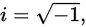what is the multiplicative inverse of 5- i?

A. 5 + i
B.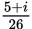C.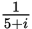D.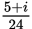E.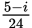4. What is the value of x: logx125 = 3?

A. 5
B. 41.7
C. 122
D. 128
E. 375

5. Givenf(x) = 7x + 5, what isf-1(x), the inverse function?

A. f-1(x) = 7x + 5
B. f-1(x) = 5x + 7
C.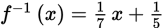D.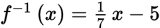E.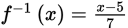6. Take 6 less than a number n. If you raise this result to the 5th power, it is equal to 32. What is the value of n?

A. 2
B. 2.07
C. 4 only
D. 4 or 8
E. 8 only

7. Take a fraction that is equivalent to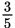and add 2 to both the numerator and the denominator. If the result is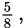what was the original fraction?

A.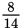B.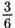C.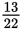D.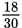E. Cannot be determined.

8. SimplIf y: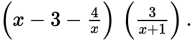A.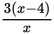B. 2x - 4
C.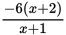D. -7
E. -12

9. When 3x3 + 2x2 + 2x + kis divided by x + 2, the remainder is 4. What is the value ofk?

A. 4
B. 24
C. 34
D. 54
E. x + 4

10. SimplIf y: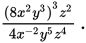A. 2x4yz2
B.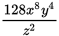C. 2x8y4z2
D.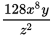E. 6y4z2

11. What is the solution to this system of equations?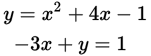A. (1,4) and (-2,5)
B. (1,-4) and(2,11)
C. (1,4) and(-2,-5)
D. (-2,7)
E. (0,1)

12. Which of the following graphs is NOT a function?

A.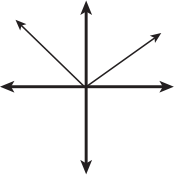B.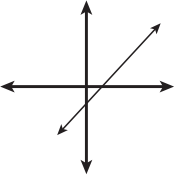C.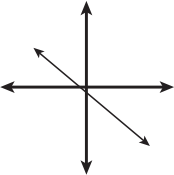D.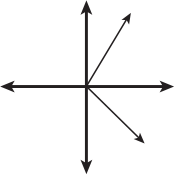E.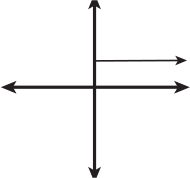13. What is the solution to this system of equations?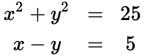A. (-5,0) only
B. (0,5) only
C. (0,-5) and(-5,0)
D. (0,5) and (5,0)
E. (0,-5) and (5,0)

14. A bus travels from point A to point B. the bus starts out going slowly and the n drives faster. Traffic is the n at a total standstill due to an accident. the bus continues at the faster speed. the bus reaches point B. Which of the following graphs could represent the bus's distance from point A, as a function of time?

A.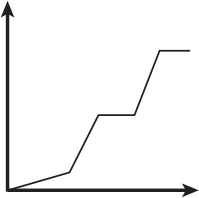B.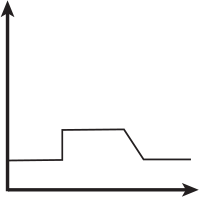C.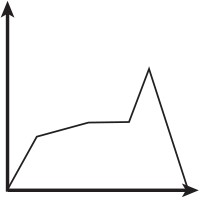D.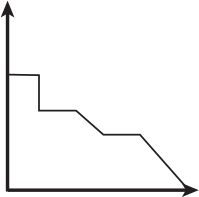E.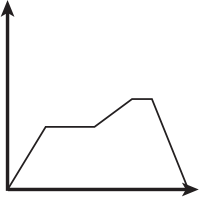15. A parallelogram has angles of measure 45 degrees and 135 degrees. the shorter side of the parallelogram measures 2.83 meters, and the o the r side is 8.83 meters. What is the area of the parallelogram, to the nearest hundredth?

A. 8.83 m2
B. 12.49 m2
C. 17.67 m2
D. 23.32 m2
E. 24.99 m2

16. A fitness club offers weights, treadmills, and aerobic classes. FIf ty members were asked which services the y used, and the results were as follows:

18members use weights and do aerobics.

25members use weights and the treadmill.

15members use the treadmill and do aerobics.

10members use all three activities.

How many members use only one of the three services?

A. 0
B. 10
C. 12
D. 18
E. 40

17. Given the equation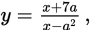what is x in terms of y and a?

A.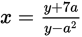B.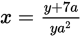C.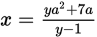D. x =y(a2 + 7a)
E. x = (y +7a)(y - a2)

18. Solve forx to the nearest hundredth: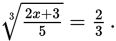A. -0.76
B. -0.69
C. -0.67
D. 0.69
E. 0.76

19. If x= y3 + 4y and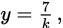what is the approximate value of x when k =21?

A. 1.37
B. 1.44
C. 1.67
D. 4.04
E. 4.11

20. Solve forx: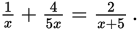A. 0.71
B. 3.57
C. 5.8
D. 25
E. 45

﻿